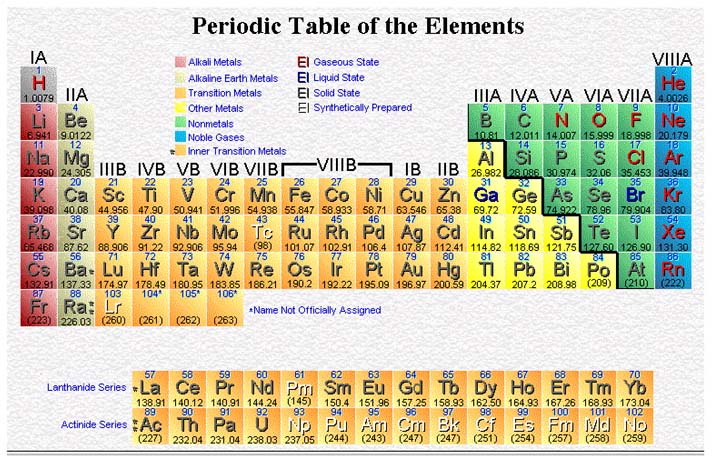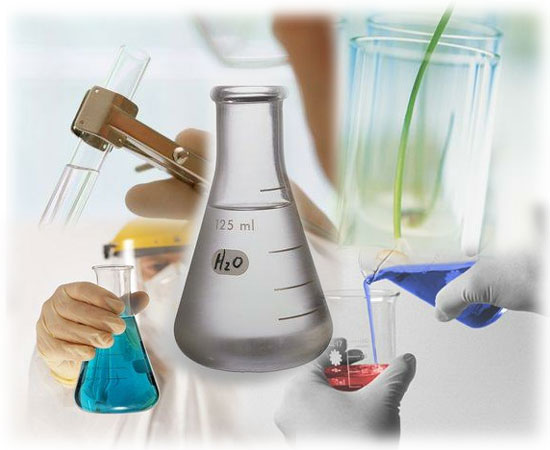CHEMISTRY Pre-AP Unit 9: Stoichiometry Chapter 11Goal: To use the mole and mole relationships to solve problems relating mass, energy, and volume.

Objectives: The student should be able to:

1. Solve problems based on mass-mass relationships in chemical reactions.

2. Solve problems based on mass-volume of solution relationships in chemical reactions.

3. Solve problems based on mass-energy relationships in chemical reactions

4. Calculate percentage yield in a chemical reaction.

5. Solve problems based on mass-volume of gas relationships in chemical reactions.

6. Solve problems based on volume-volume relationships for gases in chemical reactions.

7. Solve stoichiometric problems in which one reactant is a limiting reactant.

Activities:

Jan 4: Discuss Unit VIII Test, Lecture: Stoichiometry Mass-Mass, Percent Yield

Jan 5: Discuss homework, Lecture: Mass-Solution, Mass-Energy

Jan 8: Lab: Mass of Carbon Dioxide Released, Discuss homework

Jan 9: Lecture: Mass-Volume and Volume-Volume

Jan 10: Discuss homework, Lecture: Limiting Reactants

Jan 11: Discuss homework, Review for test.

Jan 12: Unit IX Test

Homework:

Jan 4: Practice Problems p359, 360, 362, 372

Jan 5: Worksheet I Stoichiometry, Prelab for Mass of Carbon Dioxide Released

Jan 8: Lab report due Jan 15

Jan 9: Worksheet II Stoichiometry

Jan 10: Worksheet Limiting Reactants

Jan 11: Review problems, Study for test.

Lecture & Lab Materials (uploaded once per week):

Mass-Mass Conversions and Percent Yield: This is the lecture that contains the Chemistry Online Tutorial Links!

Mass-Energy Conversions: This has a link to Dr. Wolfe's Gen Chem Practice Problems!